## Figures index

#### Pavol Lengvarský, Jozef Bocko, Martin Hagara

American Journal of Mechanical Engineering. 2015, 3(6), 220-224 doi:10.12691/ajme-3-6-13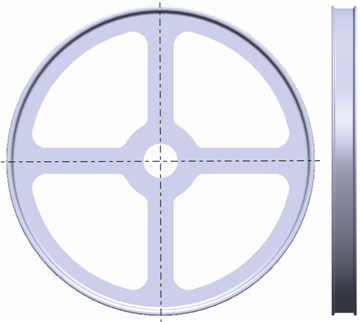• Figure 1. The pulley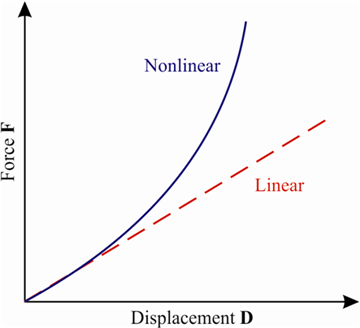• Figure 2. Linear and nonlinear functions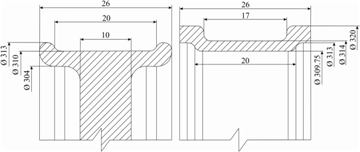• Figure 3. Design of the pulley and the enclosure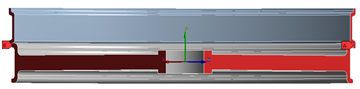• Figure 4. The finite element mesh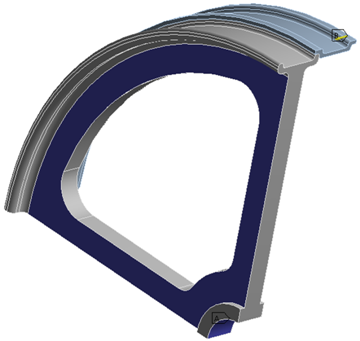• Figure 5. The surfaces with applied boundary conditions on the quarter model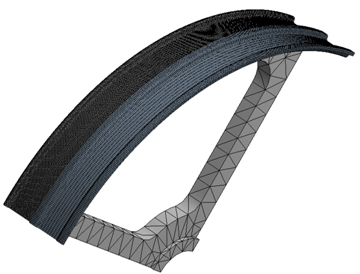• Figure 6. The finite element mesh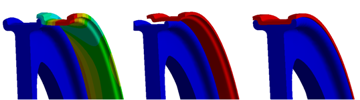• Figure 7. The process of the pressing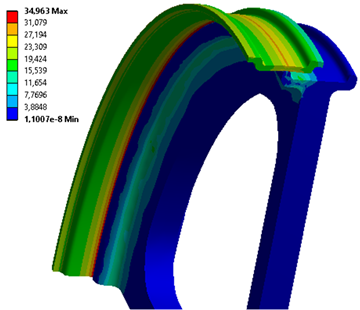• Figure 8. Stress plot of the polyethylene enclosure on the pulley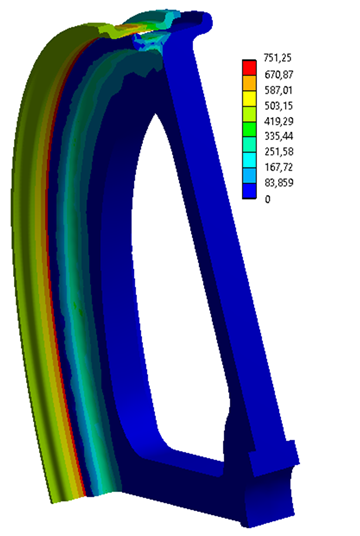• Figure 9. Stress plot of the aluminium enclosure on the pulley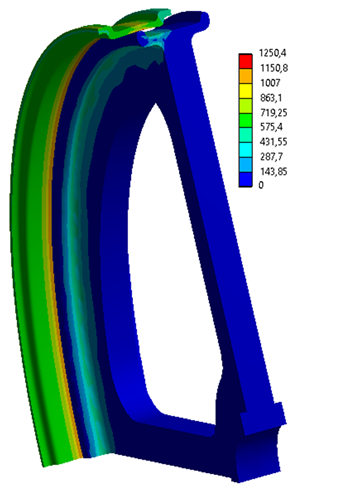• Figure 10. Stress plot of the titanium enclosure on the pulley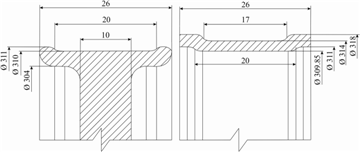• Figure 11. The first variant of both parts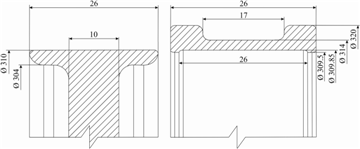• Figure 12. The second variant of both parts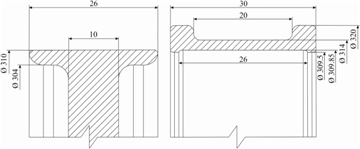• Figure 13. The third variant of both parts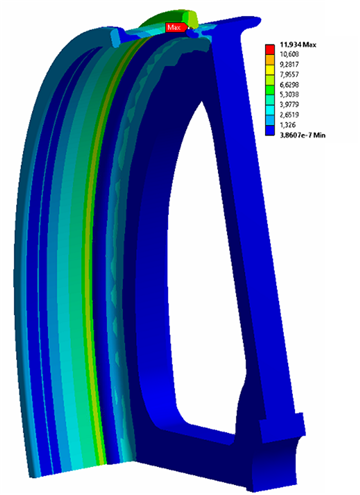• Figure 14. Stress plot of the second variant for the polyethylene enclosure on the pulley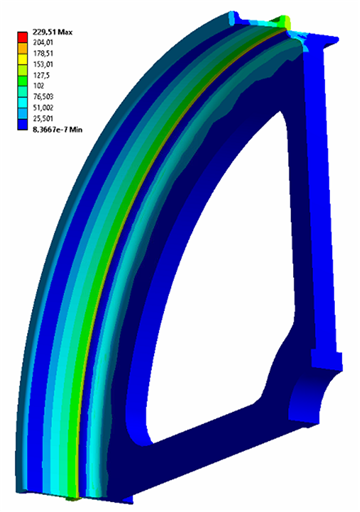• Figure 15. Stress plot of the second variant for the aluminium enclosure on the pulley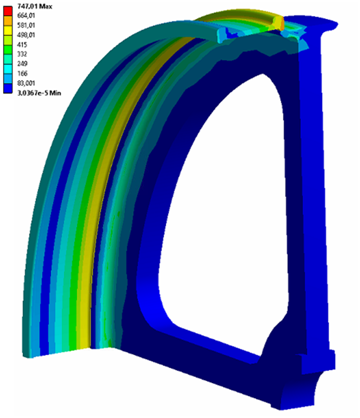• Figure 16. Stress plot of the second variant for the titanium enclosure on the pulley